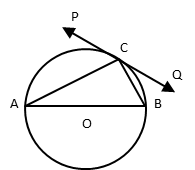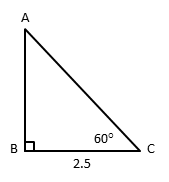# 2016 CBSE Class 10

Maths Board Paper One mark Question Video Explanations
1. #### PQ is a tangent at a point C to a circle with centre O. If AB is a diameter and ∠CAB = 30°, find ∠PCA.#### Video ExplanationAB is a diameter to the circle and O is the centre of the circle.
Join OC.
OA and OC are radii to the circle. So, OA = OC
∴ ∆ AOC is an isosceles triangle.
In an isosceles triangle, two sides and correspondingly two opposite angles are equal.
So, ∠OAC = ∠OCA = 30°

∠OCP is the angle between the tangent and the radius.
∴ ∠OCP = 90°
∠ACP = ∠OCP - ∠OCA = 90° – 30° = 60°

#### Video Explanation

k + 9, 2k - 1 and 2k + 7 are consecutive terms of an Arithmetic Progression.
Key Property: The difference between any two consecutive terms of an AP is the same.
So, (2k – 1) – (k + 9) = (2k + 7) – (2k -1)
k – 10 = 8 or k = 18

#### Video Explanationcos 60° = $$frac{BC}{AC}$ cos 60° = $\frac{1}{2}$ = $\frac{2.5}{AC}$ or AC = 5 m Length of the ladder = 5m #### Question 4: A card is drawn at random from a well shuffled pack of 52 playing cards. Find the probability of getting neither a red card nor a queen. #### Video Explanation #### Explanatory Answer Number of red cards in a pack = 26 Number of black queens = 2$Note: We only have to remove black queens as red queens would have been removed along with the 26 red cards).
Probability = $\frac{\text{Number of ways of selecting neither a red nor a queen}}{\text{Number of ways of selecting 1 card from the pack}}$
= $\frac{24}{52}$ = $\frac{6}{13}$